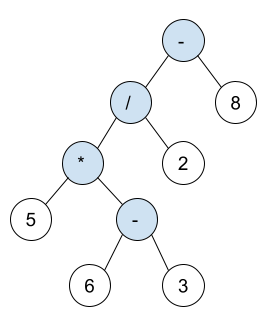New update is available. Click here to update.

# Build Binary Expression Tree From Infix Expression

Last Updated: 27 Mar, 2021
Difficulty: Moderate

## PROBLEM STATEMENT

#### Note:

``````Infix expression: The expression of the form ‘a operator b’. When an operator is in-between every pair of operands.
The expression tree is a binary tree in which each internal node corresponds to the operator and each leaf node corresponds to the operand so for example expression tree for 5 * ( 6 - 3 ) / 2 - 8 would be:
``````#### Input Format:

``````The first line of input contains an integer 'T' representing the number of test cases.

The first line of each test case contains a string S representing infix expression.
``````
##### Output Format :
``````For each test case, return the binary expression tree, whose inorder traversal is the same as 'S'.

The output for each test case is printed in a separate line.
``````

#### Note:

``````You do not need to print anything, it has already been taken care of. Just implement the given function.
``````
##### Constraints :
``````1 <= T <= 5
1 <= N <= 5000
Operands are only numbers between 0 and 9 (included).
It is guaranteed that ‘S’ is a valid infix expression.

Time limit: 1 sec
``````